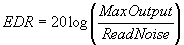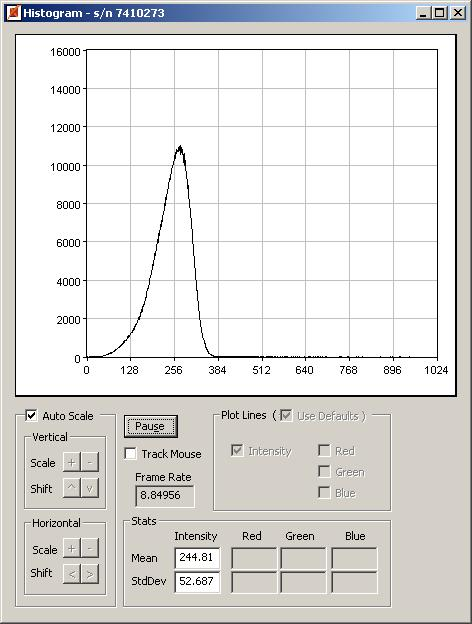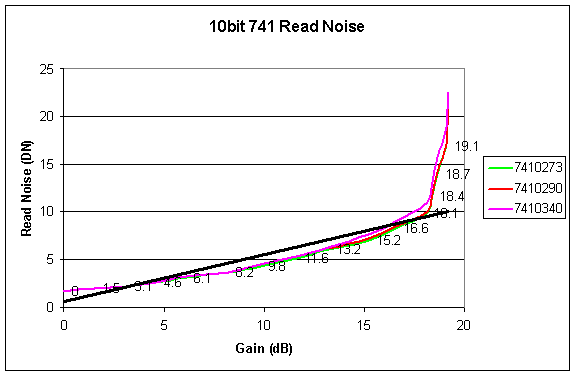Description

This tech note considers the interplay between dynamic range and camera gain for the PL-A740 series cameras.  All data shown in this report is in 10 bits.  The noise values quoted are for a 10 msec integration time.  Other integration times will yield slightly different results.  The basic principles, however, discussed in this tech note will apply.

## Dynamic Range

For a linear system, the dynamic range (DR) is equal to the signal-to-noise ratio (SNR).  DR in this context is taken as the electrical DR (EDR) defined as:where MaxOutput is the maximum usable output swing available from the camera, and not simply the output range from a camera.  ReadNoise is the uncertainty of the pixel value.  Note also that, there is a corresponding optical dynamic range, which is not presented in this tech note.

To get a sense of what MaxOutput can be, consider the scenario in the figure below.The histogram corresponds to a dark field taken from a PL-A741 camera with the following settings:

• Gain=0dB

• Flat Field Correction (FFC) optimized

Ignoring read noise, approximately 1010 Digital Numbers (DN) (=1023-13) gray levels can be utilized as MaxOutput.

Here the camera has been flat field corrected (FFC), which has the effect of reducing the spread of the histogram and the black level offset (BLO).  The gain in this case was 0dB.

Our next example shows the histogram at a higher gain (16.6dB), but with no FFC optimization.  Notice the wide spread.  This has the effect of reducing MaxOutput, and hence, the EDR.  Ignoring read noise, approximately 778 DN (=1023-245) gray levels can be utilized as MaxOutput.

Therefore, and this is important to note, even if the ReadNoise is the same at all gains, the widening histogram would result in a reduction of the EDR.  Had FFC been done at 16.6dB, the histogram spread would be reduced significantly, which would have the effect of increasing the range of utilizable gray levels, and hence, the dynamic range.The figure below shows the read noise as it varies with gain for three different PL-A741s.  Notice that for gains less than approximately 18dB, the read noise is almost linear with gain and that a knee point exists at roughly 18dB gain.  The black line shows how close the curves are to a straight line fit for gains less than 18dB.In this example, even if FFC is applied at these gains and the histogram spread is comparable to the 0dB case, the SNR will still be significantly lower due to rising read noise.  Hence for optimal SNR, try to keep the gains below 18dB.  For some applications, SNR is less important than camera responsivity.  For these applications gain greater than 18dB may give adequate results.

## Selecting the “Best” Gain for a Particular Application

In an application where there is enough light available for a camera to reach its maximum output (1023DN, for a 10bit camera), that is where light starvation is not a problem, then the lowest gain setting will always result in the best EDR.  However, when light starvation is an issue, then not all the upper gray levels can be utilized which results in an effective drop in the EDR.  To optimize the EDR for a specific application the following steps should be followed:

1.Determine the gain, integration time, and brightness that will allow all of the camera’s gray levels to be utilized.  This is most easily done by looking at the histogram using a representative light level and exposure time, and then adjusting the gain until the upper tail of the histogram approaches 1023DN.

The exact point at which the tail is close enough to 1023DN is subjective and depends on the particular application under consideration.  The effect of the brightness is to adjust the BLO.  Note that the FFC in the next step will have the effect of the reducing spread of the histogram and so, the user may wish to have some amount of high end clipping since this will be eventually reduced.

For example, let’s say that the integration time needs to be 40ms, and the lighting is such that only approximately 20% of the lower end of the histogram is used.  Increasing the gain to 11.6dB will allow close to all of the gray levels to be used.

2. Save the camera settings in Channel 1 and perform an FFC at this setting.  The Save Camera Settings command is found in the Camera menu.  The effect of FFC will reduce the BLO and the histogram spread, and thus, increasing the gray levels that are utilizable.3. Continuing on with the previous example, the camera settings at 11.6dB would be saved. These settings would  then be used by the FFC Field Tool to perform the FFC calibration.

4. At this point, the camera should be optimized for maximum SNR.  Some iterations may be required, the basic steps shown above are the ones to follow.

The Pixelink support team can help users quickly optimize the camera settings for a particular user application.  Please email us at pixelink.support@ametek.com.